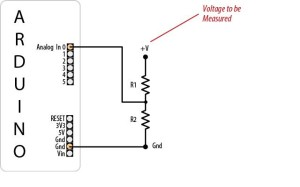Since we are involved in off grid solar power systems, we have a need to monitor battery voltage. The Arduino can do this easily with a simple voltage divider. With some simple mods, we can control loads, generators, or notifications based on battery voltage.

To read a maximum of 20vdc, R1 should be 3k ohm, R2 should be 1k ohm, and the code would be as follows:

/*
DisplayMoreThan5V sketch
prints the voltage on analog pin to the serial port
Do not connect more than 5 volts directly to an Arduino pin.
*/

const int referenceVolts = 5; // the default reference on a 5-volt board
//const float referenceVolts = 3.3; // use this for a 3.3-volt board

const int R1 = 3000; // value for a maximum voltage of 20 volts
const int R2 = 1000;
// determine by voltage divider resistors, see text
const int resistorFactor = 255 / (R2/(R1 + R2));
const int batteryPin = 0; // +V from battery is connected to analog pin 0

void setup()
{
Serial.begin(9600);
}

void loop()
{
int val = analogRead(batteryPin); // read the value from the sensor
float volts = (val / resistorFactor) * referenceVolts ; // calculate the ratio
Serial.println(volts); // print the value in volts
}

Sursa articol :http://arduinotronics.blogspot.ro/2011/03/monitoring-voltage-of-dc-battery-supply.html